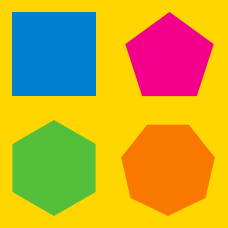Geometry

# Regular Polygons: Level 4 Challenges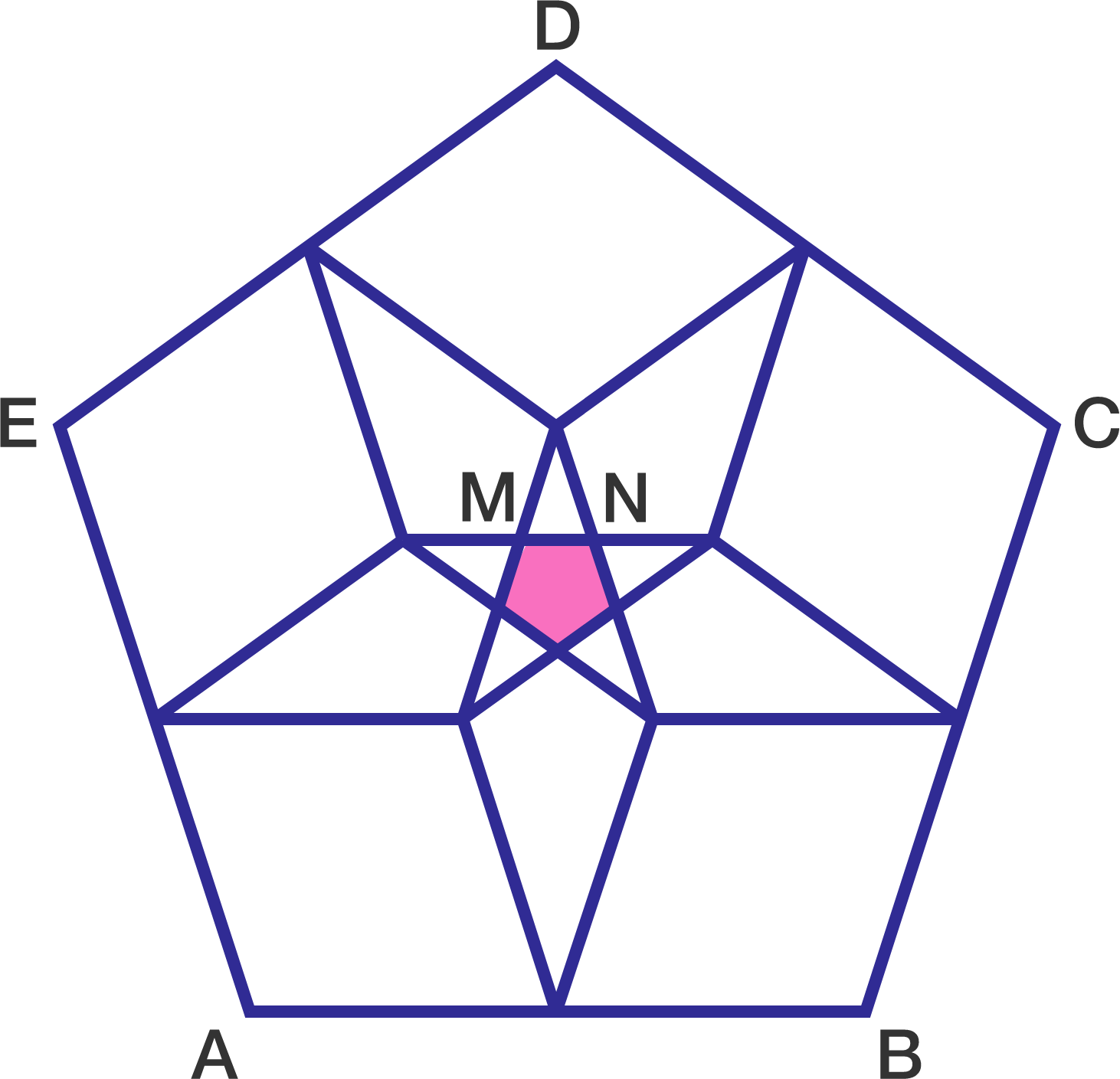Inside a regular pentagon $ABCDE$, construct 5 more regular pentagons of side length $\dfrac{1}{2}AB$. The part of the overlapping of these pentagons yields another regular pentagon of side length $MN$.

Let $r=\dfrac{AB}{MN}$. Find $\displaystyle \left \lfloor 1000r \right \rfloor$.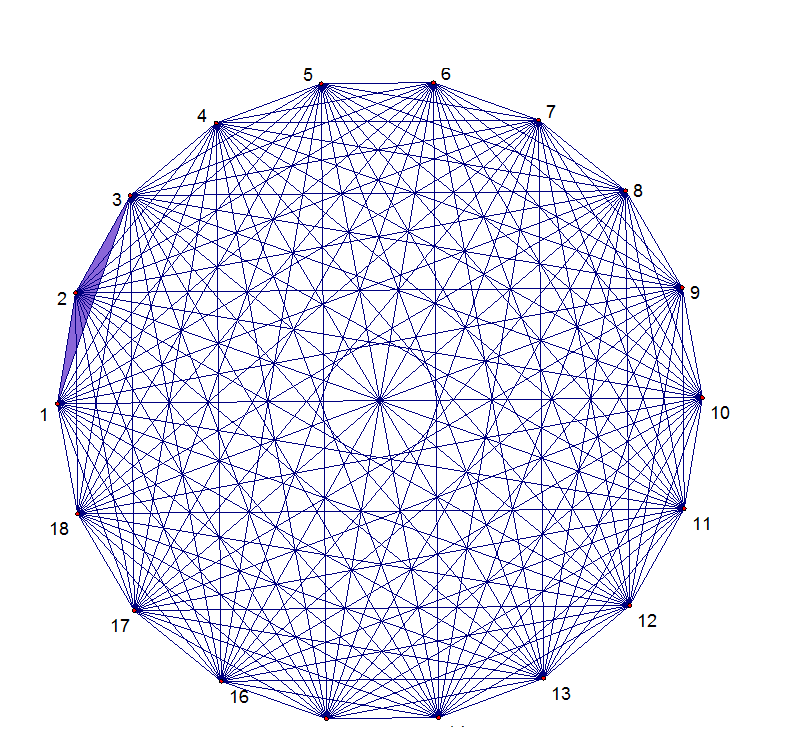Above shows a 18-sided regular polygon. How many obtuse triangles are there formed by 3 vertices?

###### Try part 1.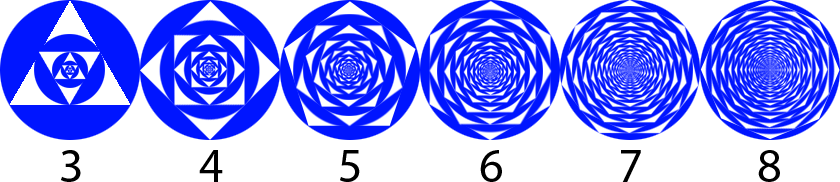The $n^{\text{th}}$ figure in the above sequence is constructed by the following procedure:

1. Draw a blue disc of radius $\displaystyle\sqrt{\frac{2016}{\pi}}$
2. Remove a regular $n$-gon area from the (smallest) disc
3. Inscribe a blue disc inside the empty $n$-gon space
4. Repeat steps 2-4

Let $A_n$ be the total blue area of the $n^{\text{th}}$ figure in the sequence.

Compute $\displaystyle\lim_{n\to\infty}A_n$.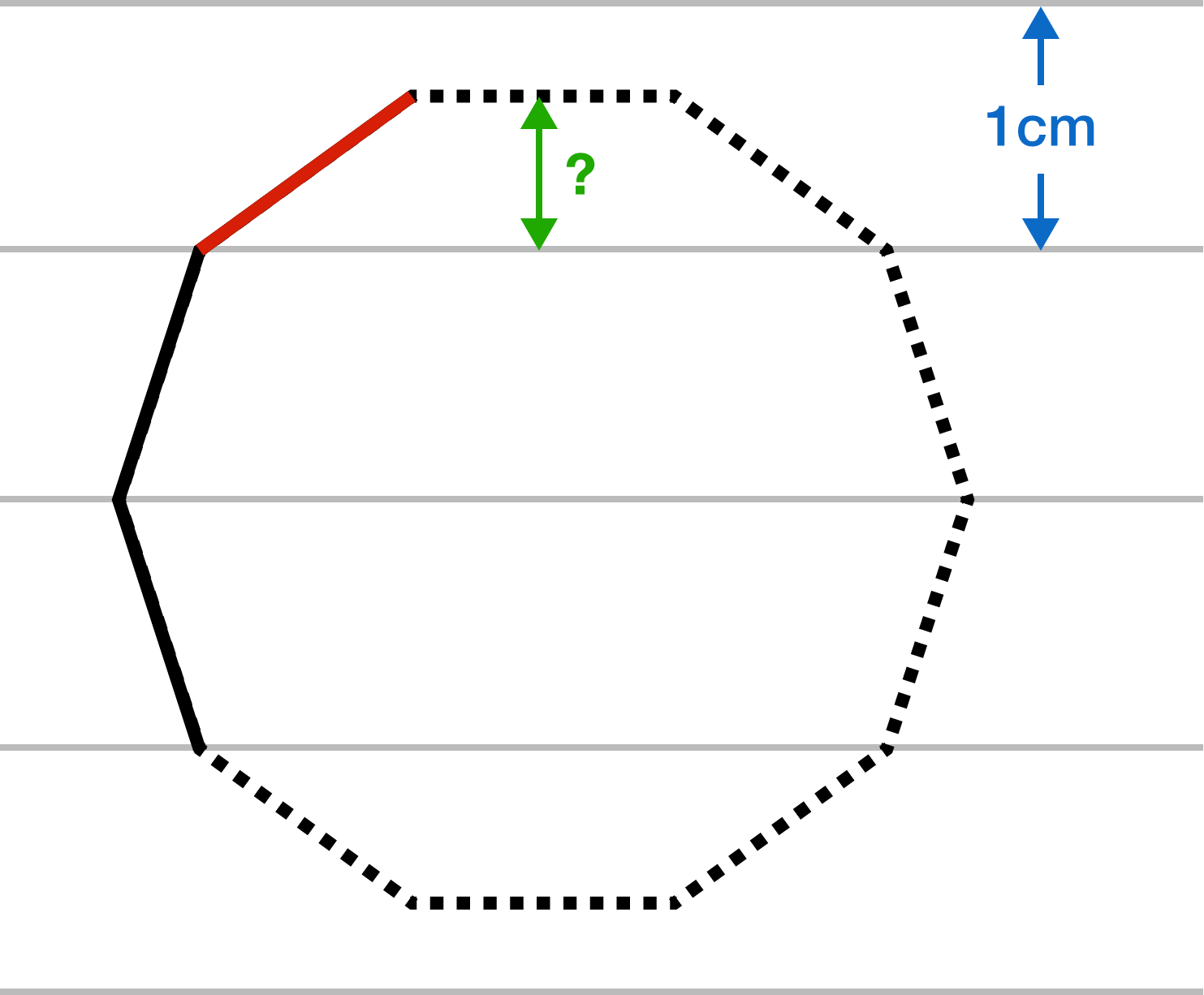It is not easy to draw a regular decagon without tools.

On a piece of writing paper (with equally spaced lines), I am trying to draw a regular decagon, as shown above. I started by drawing two sides so that their vertical extent is precisely 1 unit of the paper (black lines).

Now I want to draw the next side (red line), and I wonder how far it will extend vertically. To 3 decimal places, what is the distance marked with a question mark?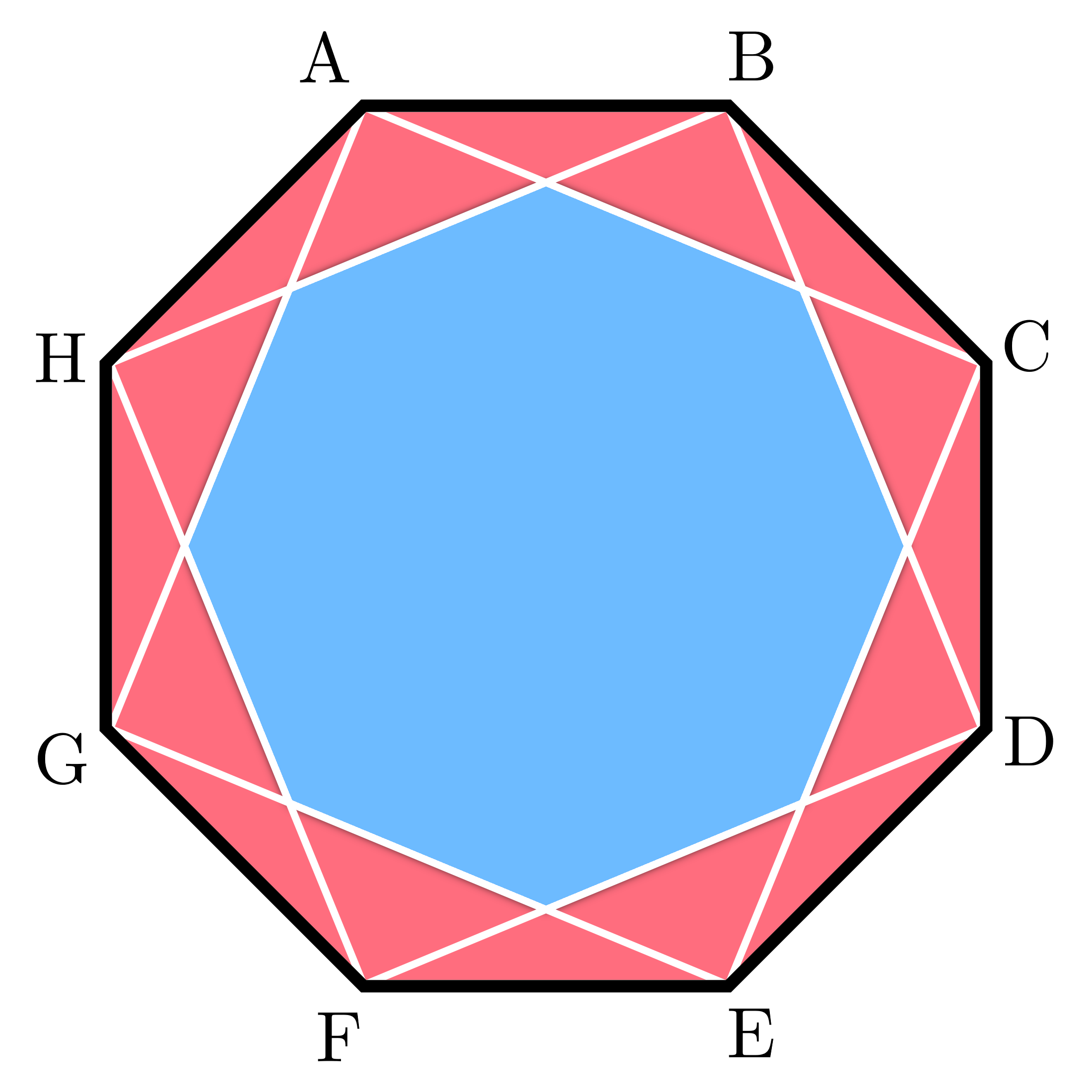A regular octagon $ABCDEFGH$ has squares $ACEG$ and $BDFH$ inscribed in it. These squares form a smaller octagon as shown.

Let the the area of octagon $ABCDEFGH$ be $A_L$ and the area of the smaller octagon be $A_S$. Then for some integers $a$ and $b$, where $b$ is square-free, $\large \dfrac{A_S}{A_L}=a-\sqrt{b}.$ Find $a+b$.

×